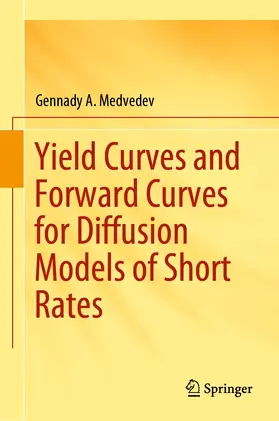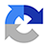# MedvedevYield Curves and Forward Curves for Diffusion Models of Short Rates

1. Auflage 2019, 230 Seiten, Gebunden, Book, Format (B × H): 160 mm x 241 mm, Gewicht: 553 g
ISBN: 978-3-030-15499-8
Verlag: Springer-Verlag GmbH

## MedvedevYield Curves and Forward Curves for Diffusion Models of Short Rates

This book is dedicated to the study of the term structures of the yields of zero-coupon bonds. The methods it describes differ from those usually found in the literature in that the time variable is not the term to maturity but the interest rate duration, or another convenient non-linear transformation of terms. This makes it possible to consider yield curves not only for a limited interval of term values, but also for the entire positive semiaxis of terms.

The main focus is the comparative analysis of yield curves and forward curves and the analytical study of their features. Generalizations of yield term structures are studied where the dimension of the state space of the financial market is increased. In cases where the analytical approach is too cumbersome, or impossible, numerical techniques are used.

This book will be of interest to financial analysts, financial market researchers, graduate students and PhD students.

Research

## Weitere Infos & Material

Preface.- Introduction.- 1.The processes of short-term interest rates and their probability densities.- 2.The term structure of interest rates.- 3.The Vasicek model.- 4.The Cox-Ingersoll-Ross model.- 5.The Duffie-Kan one-factor model.- 6.The Duffie-Kan two-factor models.- 7.The three-factor models.- 8.Another version of the term to maturity variable.- 9.The Nelson-Siegel-Svensson no-arbitrage yield curve model.- 10.Quadratic models of yield in a risk-neutral world.- 11.Polynomial models of yield term structure.- References.

Gennady A. Medvedev is a professor of physical and mathematical sciences at the Belarusian State University. His research interests are in applied statistical analysis and stochastic financial mathematics. He is the author of 11 monographs and 16 textbooks.

## Ihre Fragen, Wünsche oder Anmerkungen

Ihre Nachricht*
Wie möchten Sie kontaktiert werden?
Anrede*
Titel
Vorname*
Nachname*